# Matrix game

A two-person zero-sum game in which each player possesses only a finite number of pure strategies. If player I possessesstrategies and player II possessesstrategies, then the matrix game can be given by an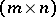-matrix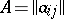, where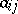,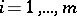,, is the payoff of player I if (s)he chooses strategywhile player II chooses strategy. According to the general optimality principle in two-person zero-sum games (see also Minimax principle), player I tends to choose a strategy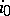on which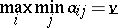is attained, whereas player II tends to choose a strategyon which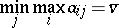is attained. If, then the pair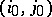is called a saddle point (cf. Saddle point in game theory) of the game; the numberis called the value of the game, and the strategies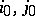are optimal pure strategies. If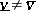(i.e. a pure strategy solution does not exist), then always. In this case one must seek the optimal strategies of the players among their mixed strategies (cf. Strategy (in game theory)). Let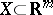(respectively,) be the set of mixed strategies of player I (respectively, player II). Then players I and II will tend to choose the strategies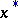and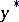on which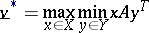andare attained, respectively (the superscript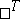denotes transposition). The main theorem in the theory of matrix games (von Neumann's minimax theorem) asserts that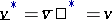, i.e. for every matrix game there are optimal mixed strategiesand a valueof the game.

For the numerical solution of matrix games (i.e. for finding optimal strategies and the value of the game) one most frequently uses the fact that the problem of solving a matrix game can be reduced to a linear programming problem. A less efficient approach is the Brown–Robinson iterative method, which amounts to "playing" fictitiously the matrix game so that at each step the players choose the best pure strategies under an "accumulated" mixed strategy of the opponent. Matrix games in which one of the players has only two strategies are readily solved using graphical methods.

Matrix games serve as mathematical models of many of the simplest conflict situations in the areas of economics, mathematical statistics, war science, and biology. In applications, the role of one of the players is sometimes assigned to "nature" , by which one understands the totality of external circumstances which are unknown to the decision maker (the other player).